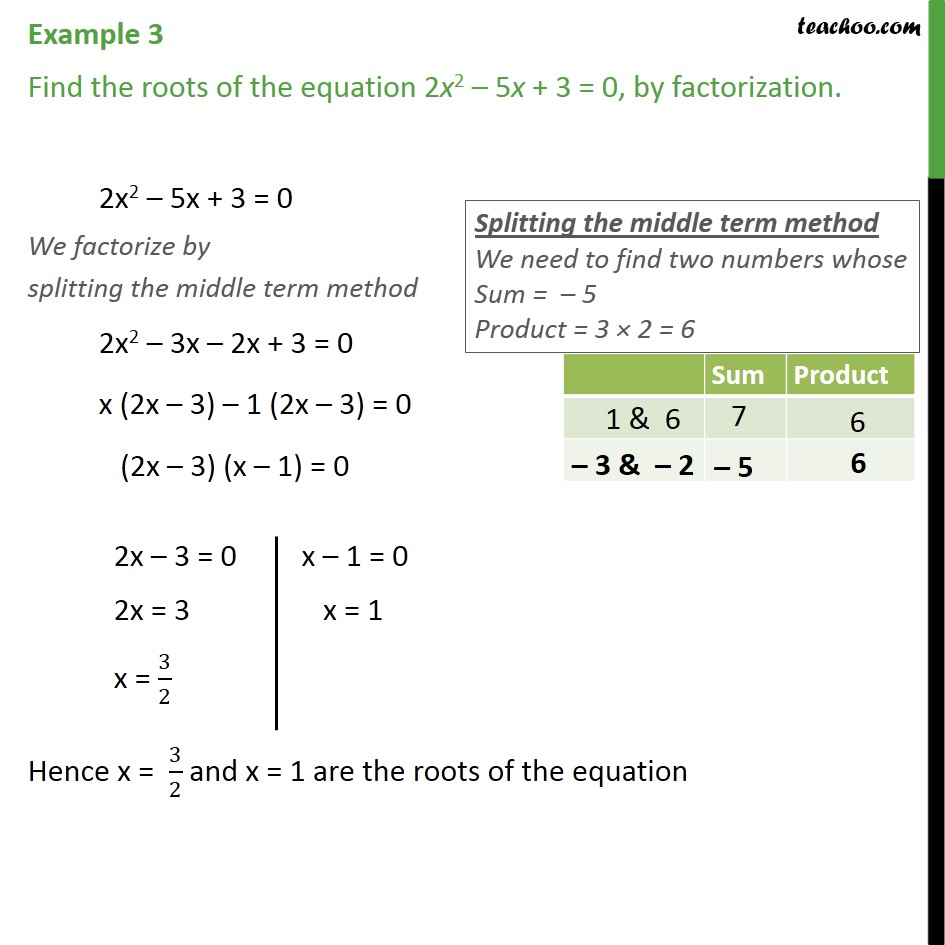1. Chapter 4 Class 10 Quadratic Equations (Term 2)
2. Concept wise
3. Solving by Splitting the middle term - Equation given

Transcript

Example 3 Find the roots of the equation 2x2 – 5x + 3 = 0, by factorization. 2x2 – 5x + 3 = 0 We factorize by splitting the middle term method 2x2 – 3x – 2x + 3 = 0 x (2x – 3) – 1 (2x – 3) = 0 (2x – 3) (x – 1) = 0 Hence x = 3/2 and x = 1 are the roots of the equation